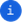# Statistics

Read statistics for a list of numbers.

#### Input Fields

• List (list of objects): list of numbersClick on an empty cell in each list to add a list item. A cell can contain only one list item. Statistics will only be computed for list items that are numbers.

#### Output Fields

• Sum: Sum of all the numbers in the list
• Average: Average of all the numbers in the list
• Median: Median value for the numbers in the list, the value for which half the numbers are higher or lower
• Mode: Number which appears most often in the list
• Variance: Degree of spread in the list of numbers; variance is calculated by taking the average of squared deviations from the mean.
• Standard Deviation: Standard deviation for the list; standard deviation tells you how far each value lies from the mean; it's the square root of variance.
• Minimum: Minimum; the lowest number in the list.
• Maximum: Maximum; the highest number in the list.
• Count: Number of values in the list

#### Example

For the eight values in this list:

1,2,4,1,8,16,32,1

The resulting statistics are:

• Sum: 65
• Average: 8.12
• Median: 3
• Mode: 1
• Variance: 119.84
• Standard Deviation: 10.947113122384627
• Min: 1
• Max: 32
• Count: 8